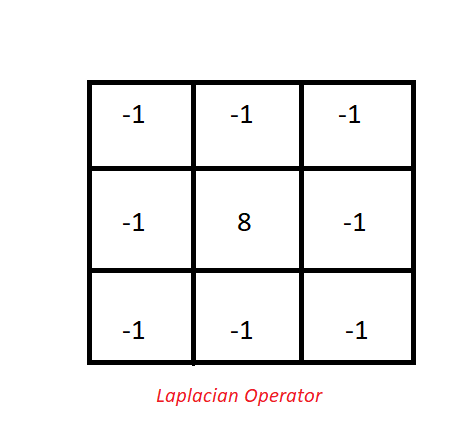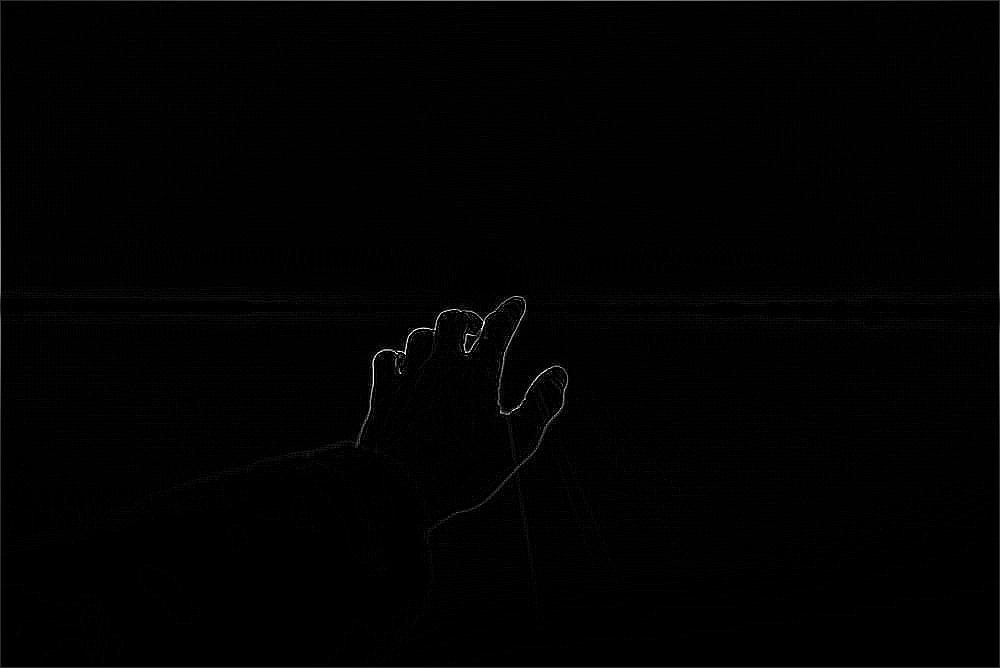# Python – Edge Detection using Pillow

Edge Detection, is an Image Processing discipline that incorporates mathematics methods to find edges in a Digital Image. Edge Detection internally works by running a filter/Kernel over a Digital Image, which detects discontinuities in Image regions like stark changes in brightness/Intensity value of pixels.

There are two forms of edge detection:

• Search Based Edge detection (First order derivative)
• Zero Crossing Based Edge detection (Second order derivative)

Some of the commonly known edge detection methods are:

• Laplacian Operator or Laplacian Based Edge detection (Second order derivative)
• Canny edge detector (First order derivative)
• Prewitt operator (First order derivative)
• Sobel Operator (First order derivative)

We would be implementing a Laplacian Operator in order to incorporate Edge detection in one of our later examples. For this purpose, we will be using `pillow` library. To install the library, execute the following command in the command-line :

```pip install pillow
```

NOTE:- Several Linux distributions tend to have Python and Pillow preinstalled onto them.

There are two ways in which we would be implementing Edge detection on our images. In the first method we would be using an inbuilt method provided in the pillow library (`ImageFilter.FIND_EDGES`) for edge detection. In the second one we would be creating a Laplacian Filter using `PIL.ImageFilter.Kernel()`, and then would use that filter for edge detection.

LAPLACIAN KERNEL:-SAMPLE IMAGE:-Method 1:

 `from` `PIL ``import` `Image, ImageFilter ` ` `  ` `  `# Opening the image (R prefixed to string ` `# in order to deal with '\' in paths) ` `image ``=` `Image.``open``(r``"Sample.png"``) ` ` `  `# Converting the image to greyscale, as edge detection  ` `# requires input image to be of mode = Greyscale (L) ` `image ``=` `image.convert(``"L"``) ` ` `  `# Detecting Edges on the Image using the argument ImageFilter.FIND_EDGES ` `image ``=` `image.``filter``(ImageFilter.FIND_EDGES) ` ` `  `# Saving the Image Under the name Edge_Sample.png ` `image.save(r``"Edge_Sample.png"``)`

Output (Edge_Sample.png):Explanation:-

Firstly we create an image object of our image using `Image.open()`. Then we convert the Image color mode to greyscale, as the input to the Laplacian operator is in greyscale mode (in general). Then we pass the image onto `Image.filter()` function by specifying `ImageFilter.FIND_EDGES` argument, which in turns runs a edge detection kernel on top of the image. The output of the above function results in an image with high intensity changes (edges) in shades of white, and rest of the image in black color.

Method 2:

 `from` `PIL ``import` `Image, ImageFilter ` ` `  `img ``=` `Image.``open``(r``"sample.png"``) ` ` `  `# Converting the image to greyscale, as Sobel Operator requires ` `# input image to be of mode Greyscale (L) ` `img ``=` `img.convert(``"L"``) ` ` `  `# Calculating Edges using the passed laplican Kernel ` `final ``=` `img.``filter``(ImageFilter.Kernel((``3``, ``3``), (``-``1``, ``-``1``, ``-``1``, ``-``1``, ``8``, ` `                                          ``-``1``, ``-``1``, ``-``1``, ``-``1``), ``1``, ``0``)) ` ` `  `final.save(``"EDGE_sample.png"``) `

Output (EDGE_sample.png):Explanation:-

Firstly we create an image object of our image using `Image.open()`. Then we convert the Image color mode to greyscale, as the input to the Laplacian operator is in greyscale mode (in general). Then we pass the image onto `Image.filter()` function by specifying our operator/Kernel inside the function as an argument. The Kernel is specified by using `ImageFilter.Kernel((3, 3), (-1, -1, -1, -1, 8, -1, -1, -1, -1), 1, 0))` which create a 3 X 3 Kernel (3 pixel Wide and 3 pixel long) with the values (-1, -1, -1, -1, 8, -1, -1, -1, -1) (as stated in the Laplacian Kernel image). The `1` argument (after the kernel) stands for the Scale value, which divides the final value after each kernel operation, therefore we set that value to 1 as we don’t want any division to our final value. The `0` argument (after the Scale value) is the offset which is added after the division by Scale value. We have set that value to 0 as we don’t want any increment to the final intensity value after the Kernel Convolution. The output of the above function results in an image with high intensity changes (edges) in shades of white, and rest of the image in black color.

Both the programs yielded the same result. The reason for which being the fact that the inbuilt function `ImageFilter.FIND_EDGE` uses a 3 X 3 sized Laplacian Kernel/Operator internally. Due to which we ended up with identical results. The benefit of using a Kernel instead of relying on inbuilt functions is that we can define kernels according to our needs, which may/may not be in the library. Such as we can create a Kernel for Blurring, Sharpening, Edge detection (using other Kernels) etc. Also, I intentionally chose the Laplacian so that we can maintain consistency in results.

Benefits of using Laplacian:-
Fast and decent results. Other common edge detectors like Sobel (first order derivative) are more expensive on computation, as they require finding Gradients in two directions and then Normalizing the results.

Drawbacks of using laplacian:-
Convolving with Laplacian Kernel leads to a lot of noise in the output. This issue is resolved by other Edge Detection methods such as Sobel, Perwitt Operator etc. As they have a built-in Gaussian Blur Kernel in them. Which reduces the noise obtained from the input image. They also lead to more accurate edge detection, due to the higher computation involved into finding them.

My Personal Notes arrow_drop_upCheck out this Author's contributed articles.

If you like GeeksforGeeks and would like to contribute, you can also write an article using contribute.geeksforgeeks.org or mail your article to contribute@geeksforgeeks.org. See your article appearing on the GeeksforGeeks main page and help other Geeks.

Please Improve this article if you find anything incorrect by clicking on the "Improve Article" button below.

Article Tags :

Be the First to upvote.

Please write to us at contribute@geeksforgeeks.org to report any issue with the above content.